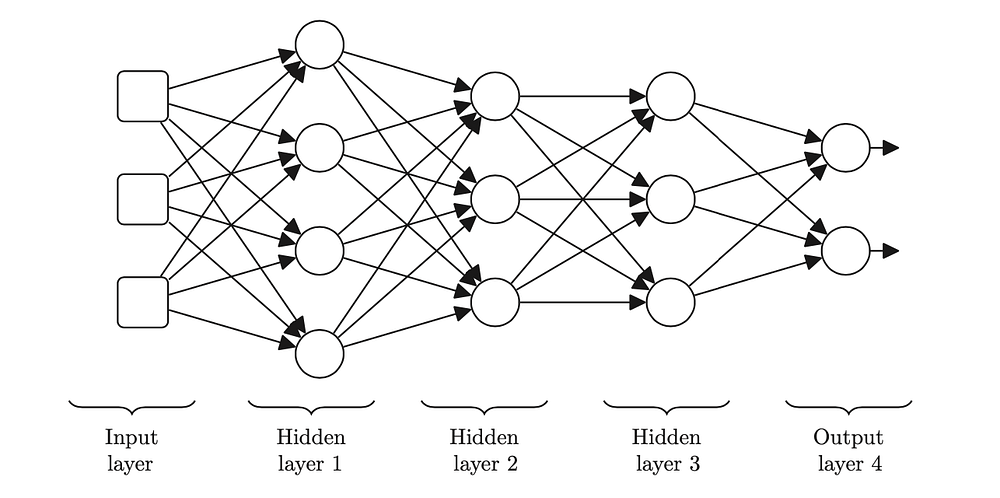# Building Blocks of Neural Networks

Original article can be found here (source): Deep Learning on Medium# Building Blocks of Neural Networks

The term deep learning describes a family of neural network models that have multiple layers of simple information processing programs, known as neurons, in the network ( John. D.Kelleher — Deep Learning)

A neural network is inspired by the structure of human brain. Human brain, according to scientists, is consist of billions neurons that transmit signals to each other.

When we build a deep learning model, or any model, whether its complex or simple algorithm we always try to encapsulate real world into mathematical formulas. So in other words, a neural networks are mathematical models.

A picture above is common neural network’s structure. It consist of input layers, hidden layer(s), and output layer. Number of hidden layers vary, and their quantity depends on the problem you’re working on. Too many hidden layers makes a model very complex, and it is very likely that it will overfit. At the same time if you have not enough layers your model will not perform well and it may take a lot of time to compute if you have a large dataset.

At each layer complex functions get computed. Those functions called activation functions. Activation functions decide whether node gets activated or not. This adds non-linearity to the model. There are many activation functions but these are common ones: sigmoid function, ReLU, tanh, leaky ReLU and etc.

In conclusion, we can say that deep learning models are just a large number of simple processing units that are composed together, they work together to learn and recognize patterns from large datasets.